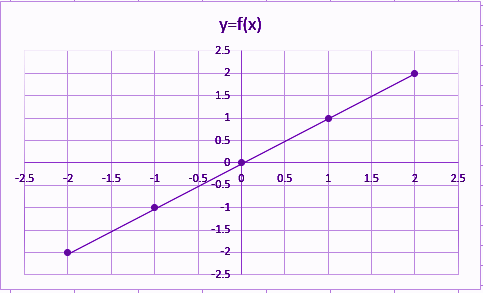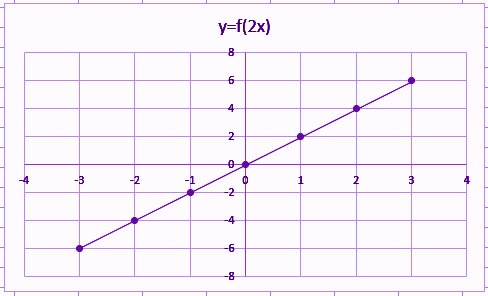# Identity Function

The identity function is a function which returns the same value, which was used as its argument. It is also called an identity relation or identity map or identity transformation. If f is a function, then identity relation for argument x is represented as f(x) = x, for all values of x. In terms of relations and functions, this function f: P → P defined by b = f (a) = a for each a ϵ P, where P is the set of real numbers. Both the domain and range of function here is P and the graph plotted will show a straight line passing through the origin.

## Identity Function Definition

Let R be the set of real numbers. Thus, the real-valued function f : R → R by y = f(a) = a for all a ∈ R, is called the identity function. Here the domain and range (codomain) of function f are R. Hence, each element of set R has an image on itself. The graph is a straight line and it passes through the origin. The application of this function can be seen in the identity matrix.

Mathematically it can be expressed as;

 f(a) = a ∀ a ∈ R

Where a is the element of set R.

For example, f(2) = 2 is an identity function.

In set theory, when a function is described as a particular kind of binary relation, the identity function is given by the identity relation or diagonal of A, where A is a set.

### Identity Function Graph

If we plot a graph for identity function, then it will appear to be a straight line. Let us plot a graph for function say f(x) = x, by putting different values of x.

 x -2 -1 0 1 2 f(x) = y -2 -1 0 1 2

Now as you can see from the above table, the values are the same for both x-axis and y-axis. Hence, let us plot a graph based on these values.So, from the above graph, it is clear that the identity function gives a straight line in the xy-plane.

Let us solve some examples based on this concept.

### Identity Function Example

Q.1: Prove f(2x) = 2x is an identity function.

Solution: Given, f(2x) = 2x

Let us put the values of x in the given function.

If x = 1, then;

f(2(1)) = 2(1) ⇒ f(2) = 2

If x = 2, then;

f(2(2)) = 2(2) ⇒ f(4) = 4

If x = 3, then;

f(2(3)) = 2(3) ⇒ f(6) = 6

If x = 0, then;

f(2(0)) = 2(0) ⇒ f(0) = 0

Let us try with some negative values of x.

If x =-1, then;

f(2(-1)) = 2(-1) ⇒ f(-2) = -2

If x = -2, then;

f(2(-2)) = 2(-2) ⇒ f(-4) = -4

If x = -3, then;

f(2(-3)) = 2(-3) ⇒ f(-6) = -6

Let us draw a table for all values of x.

 x -3 -2 -1 0 1 2 3 y=f(x) -6 -4 -2 0 2 4 6

Let us draw the graph for these values.You can see from the above graph. The function f(2x) = 2x plots a straight line, hence it is an identity function.

### Properties of Identity Function

• It is a linear operator in case of application of vector spaces.
• For positive integers, it is a multiplicative function.
• For m-dimensional vector space, it is expressed as identity matrix Im.
• In topological space, this function is always continuous.

#### 1 Comment

1. Vaibhav sanghani

I am new one to byjus
So please give me instructions for it